# Matrices Class 12 Notes Mathematics

Matrices Class 12 Notes Mathematics in PDF are available for free download in myCBSEguide mobile app. The best app for CBSE students now provides Matrices class 12 Notes latest chapter wise notes for quick preparation of CBSE board exams and school-based annual examinations. Class 12 Mathematics notes on chapter 3 Matrices are also available for download in CBSE Guide website.

## Matrices Class 12 Notes Mathematics

Download CBSE class 12th revision notes for chapter 3 Matrices in PDF format for free. Download revision notes for Matrices class 12 Notes and score high in exams. These are the Matrices class 12 Notes prepared by team of expert teachers. The revision notes help you revise the whole chapter 3 in minutes. Revision notes in exam days is one of the best tips recommended by teachers during exam days.

## CSBE Class 12 Mathematics Chapter 3 Matrices

• A matrix is an ordered rectangular array of numbers, real or complex or functions.
• A matrix having m rows and n columns is called a matrix of order m × n.
• Column matrix: A matrix with one column is denoted by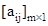.
• Row matrix: A matrix with one row is denoted by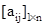.
• Square matrix: An m × n matrix is a square matrix if m = n.
• Diagonal matrix: A =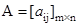is a diagonal matrix if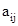=0, when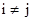• Scalar matrix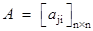is a scalar matrix if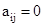when  i ≠  j,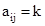(k is some constant), when I=j.
• Identity matrix: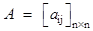is an identity matrix, if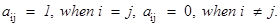• Zero matrix: A zero matrix has all its elements as zero.
• Equality of two matrices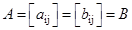if (i) A and B are of same order, (ii) for all possible values of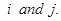• Scalar multiplication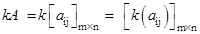Also       – A = (–1)A

• A – B = A + (–1) B

A + B = B + A

(A + B) + C = A + (B + C), where A =  B =  and C =  are of same order.

• k(A + B) = kA + kB, where A and B are of same order, k is constant.
• (k + l ) A = kA + lA, where k and l are constant.
•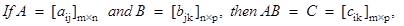where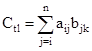• (i)      A(BC) = (AB)C,

(ii)     A(B + C) = AB + AC,

(iii)    (A + B)C = AC + BC

•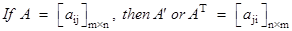• (i) (A′)′ = A, ·   (ii) (kA)′ = kA′, ·   (iii) (A + B)′ = A′ + B′, ·   (iv) (AB)′ = B′A′
• Symmetric matrix: A is a symmetric matrix if A′ = A.
• Skew-aymmetric matrix: A is a skew symmetric matrix if A′ = –A.
• Any square matrix can be represented as the sum of a symmetric and a skew symmetric matrix. In fact, A = (A + A’) + (A – A’), where (A + A’) is a symmetric matrix and (A – A’) is a skew-symmetric matrix.
• Equivalent matrices: Two matrices A and B are equivalent that is, A – B is A is obtained from the other by a sequence of elementary operations. Elementary operations of a matrix are as follows:

(i)      or  (interchange rows or columns)

(ii)     or

(iii)    or

• If A and B are two square matrices such that AB = BA = I, then B is the inverse matrix of A and is denoted by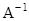and A is the inverse of  B.
• Inverse of a square matrix, if it exists, is unique.

## CBSE Class 12 Revision Notes and Key Points

Matrices class 12 Notes Mathematics. CBSE quick revision note for class-12 Chemistry Physics Math’s, Biology and other subject are very helpful to revise the whole syllabus during exam days. The revision notes covers all important formulas and concepts given in the chapter. Even if you wish to have an overview of a chapter, quick revision notes are here to do if for you. These notes will certainly save your time during stressful exam days.

To download Matrices class 12 Notes Mathematics, sample paper for class 12 Physics, Chemistry, Biology, History, Political Science, Economics, Geography, Computer Science, Home Science, Accountancy, Business Studies and Home Science; do check myCBSEguide app or website. myCBSEguide provides sample papers with solution, test papers for chapter-wise practice, NCERT Matrices, NCERT Exemplar Matrices, quick revision notes for ready reference, CBSE guess papers and CBSE important question papers. Sample Paper all are made available through the best app for CBSE students and myCBSEguide website.Test Generator

Create question paper PDF and online tests with your own name & logo in minutes.myCBSEguide

Question Bank, Mock Tests, Exam Papers, NCERT Solutions, Sample Papers, Notes